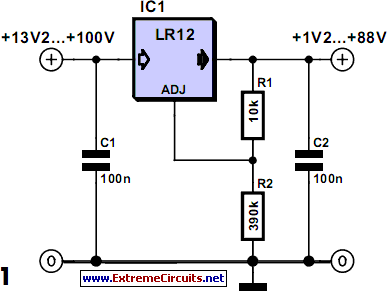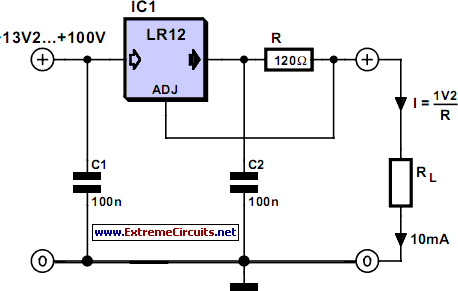# Learning Electronics

Learn to build electronic circuits

# 100V Regulators

Standard three-legged voltage regulator chips like the LM317 can cope with an input voltage of up to about 30 V, a few high-voltage types can handle 60 V but if that is still not sufficient for your application the company Supertex (www.super-tex.com) produce devices that can with-stand much higher input voltages. The regulator type LR8 has a maximum input voltage of 450 V and can supply an output current of 20 mA. The LR 12 has a better output current of 50 mA but with a maximum input voltage of 100 V, and the output voltage can be adjusted up to 88 V.

Circuit diagram:100V Regulators Circuit Diagram

The output voltage is defined by a potential divider chain connected between the output and the ADJ (adjust) input pin. The regulator simply changes its output voltage until the divided voltage at the ADJ input is equal to 1.2 V. The output voltage can be more precisely expressed as a function of R1 and R2 in the formula: VOUT = 1.2 V [1 + (R2/R1)] and R2 = R1 [(VOUT/1.2 V) - 1]

Circuit diagram:100V Regulators Circuit Diagram

The current through R1 and R2 must be greater than 100 µA. Just like conventional voltage regulators the LR12 can also be configured as a constant current source. Again the regulator simply adjusts its output voltage until it measures 1.2 V at the ADJ input. For a constant current of 10 mA the value of the series resistor is equal to the resistance that will produce a voltage drop of 1.2 V when 10 mA passes through it. As mentioned above the maximum output current is limited to 50 mA. A capacitor of 100 nF is necessary at the output to ensure stable regulator operation. The LR12 is available in SO-8, TO-92 and TO-252/D-PAK outlines.
Author: Gregor Kleine - Copyright: Elektor Electronics